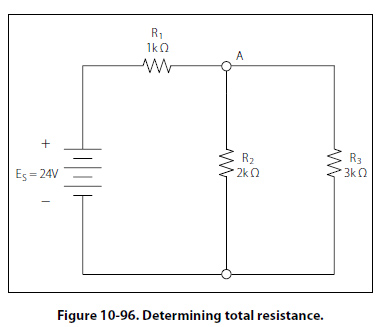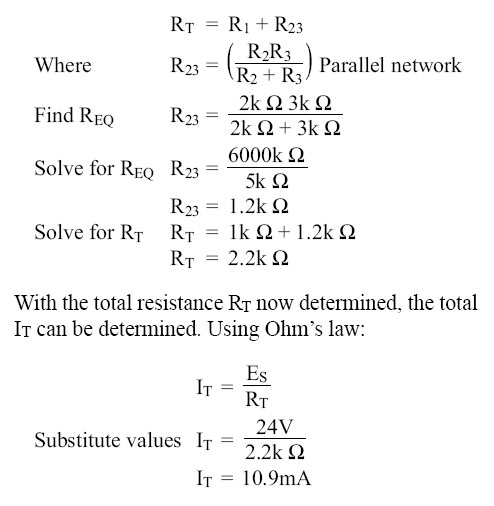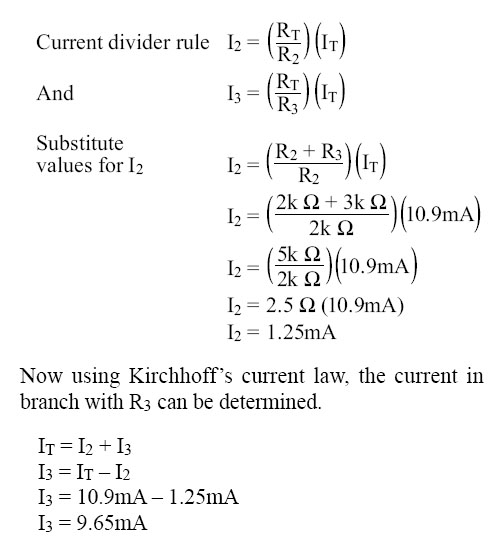Determining the Total Resistance A more quantitative example for determining total resistance and the current in each branch in a combination circuit is shown in the following example. Also refer to Figure 10-96.The first step is to determine the current at junction A, leading into the parallel branch. To determine the IT, the total resistance RT of the entire circuit must be known. The total resistance of the circuit is given as:The current through the parallel branches of R2 and R3 can be determined using the current divider rule discussed earlier in the text. Recall that:©AvStop Online Magazine                                                                                                                                                      Contact Us              Return To Books